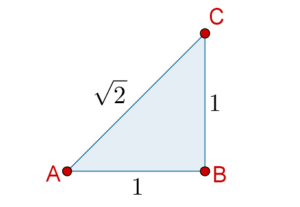# Irrational Numbers

Irrational Numbers
Go back to  'Numbers'

## Introduction:

Consider the Rational Line, that is, the set of all rational numbers plotted on a straight line. Are there any gaps in this line? Are there any points that have not been represented, or which have been left out? If we were traveling on this line, would we encounter holes, positions for which no corresponding rational numbers exist?

There do exist numbers that are not rational, which cannot be represented using integers and the four simple arithmetic operations. In other words, there are numbers that cannot be represented in the form of $$\frac{p}{q}$$ where  $${p}$$ and $${q}$$ are integers. These numbers are called Irrational Numbers.

Consider the following carefully:

1. There exist real, physical lengths that cannot be represented by rational numbers. This means that on the number line, there will be positions that cannot be assigned a rational number representation. Think about this in terms of geometry. For example, think about the rational number $$\frac{{14}}{{23}}$$. Geometrically speaking, take a unit length, divide it into 23 equal segments, and take 14 of those segments and put them together. The length you get will be $$\frac{{14}}{{23}}$$ of a unit length. However, not every length can be thought of this way. You can geometrically construct lengths to which no number of the form $$\frac{p}{q}$$ can be assigned. This is a very deep concept; take your time and reflect on it.
2. We have seen that between any two rational numbers, no matter how close, there will exist infinitely many rational numbers. However, there will be (infinitely many) gaps as well, as we have said already: the Rational Line is not continuous. It is full of gaps, and those gaps correspond to Irrational Numbers.

## What is an Irrational Number?

Irrational Numbers are the numbers that cannot be represented using integers in the $$\frac{p}{q}$$ form. The set of irrational numbers is denoted by $$\mathbb{I}$$

Some famous examples of irrational numbers are:

1. $$\sqrt 2$$ is an irrational number. The first few digits looks like this: $\sqrt 2 = 1.4142135623730950...(etc)$
2. $$\pi$$ is a famous irrational number. People have calculated Pi to over a quadrillion decimal places and still, there is no pattern. The first few digits look like this: $\pi = 3.14159265358979323...(etc)$
3. The number $$e$$ (Euler's Number) is another famous irrational number. People have also calculated $$e$$ to lots of decimal places without any pattern showing. The first few digits look like this: $e = 2.71828182845904523...(etc)$
4. The number $$\varphi$$ (Golden Ratio)  is an irrational number. The first few digits look like this: $\varphi = 1.61803398874989484820...(etc)$

Fun fact: In the ancient world, more than two thousand years ago, many mathematicians believed that every geometrical length can be represented using a rational number. In other words, it was believed that every number is rational, that is, every number has a $$\frac{p}{q}$$ representation. Rational numbers were believed to be sufficient to describe the universe. This view was held particularly strongly by members of the Pythagoras Brotherhood (the source of the Pythagoras Theorem). It, therefore, came as a strong shock to them when one of their own members discovered a number that could not be represented in the form $$\frac{p}{q}$$. He found that a valid geometrical length had no corresponding $$\frac{p}{q}$$ representation. This was such a shocking and unpleasant discovery for the Brotherhood that legend says that the discoverer of the number was thrown off a ship and drowned!

✍Note: The discoverer was Hippasus and the number we are talking about is $$\sqrt 2$$.

Consider a right-angled isosceles triangle, with the equal sides of length 1 unit. By the Pythagoras Theorem, the hypotenuse will be $$\sqrt 2$$:For a long time, mathematicians struggled to find a rational representation of $$\sqrt 2$$, until it was shown (by an unfortunate Pythagorean) that it is not a rational number at all! Thus was discovered the first known irrational number.

✍Note: Square root of any prime number is an irrational number.

## Multiplying Irrational numbers:

Does the multiplication of two irrational numbers will give you a rational or an irrational number? Let's try to understand it better by taking an example:

• $$\pi \times \pi = {\pi ^2}$$ is irrational.
• $$\sqrt 2 \times \sqrt 2 = 2$$ is rational.

✍Note: Thus, the product of two irrational numbers can either be rational or irrational.

## Solved Example:

Example 1: Which of the following is not an irrational number?

$\sqrt 2 ,{\text{ }}\sqrt 3 ,{\text{ }}\sqrt 4 ,{\text{ }}\sqrt 5$

Solution: 4 is a perfect square,i.e., $$\sqrt 4 = 2$$ which is a rational number.

Also, we know that the square root of a prime number will be an irrational number. 2, 3 and 5 are prime numbers.

So, $$\sqrt 4$$ is the required answer.Challenge: What can you say about the irrationality of circumference and area of a circle whose diameter is 6cm.

⚡Tip: $$\pi$$ is an irrational number.

## Important Topics

Numbers and Number Systems
Numbers and Number Systems
grade 9 | Questions Set 2
Numbers and Number Systems